Courses

# NCERT Exemplar - Gravitation (Part - A) Class 9 Notes | EduRev

## Class 9 : NCERT Exemplar - Gravitation (Part - A) Class 9 Notes | EduRev

The document NCERT Exemplar - Gravitation (Part - A) Class 9 Notes | EduRev is a part of the Class 9 Course Science Class 9.
All you need of Class 9 at this link: Class 9

Q.1. What is the source of centripetal force that a planet requires to revolve around the Sun? On what factors does that force depend?
Ans: The gravitational force of attraction of the Sun provides centripetal acceleration to the planet to revolve around the Sun.
F ∝ Mass  of the Sun
F ∝ Mass of the planet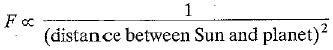Q.2. On the earth, a stone is thrown from a height in a direction parallel to the earth’s surface while another stone is simultaneously dropped from the same height. Which stone would reach the ground first and why?
Ans: Both reach the ground together because time to reach the ground depends only on vertical height.
Initial velocity in downward direction is zero and the value of 'g' is same in both cases.

Q.3. Suppose gravity of the earth suddenly becomes zero, then in which direction will the moon begin to move if no other celestial body affects it?
Ans: Moon will move along a straight line in tangential direction at the point where gravitational force ceases to exist.

Q.4. Identical packets are dropped from two aeroplanes, one above the equator and the other above the north pole, both at height h. Assuming all conditions are identical, will those packets take same time to reach the surface of the earth? Justify your answer:
Ans: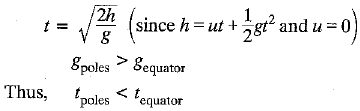Q.5. The weight of any person on the moon is about 1/6 times that on the earth. He can lift a mass of 15 kg on the earth. What will be the maximum mass, which can be lifted by the same force applied by the person on the moon?
Ans: The person can lift a mass of 15 x 6 = 90 kg on the moon by applying the same force.

Q.6. Calculate the average density of the earth in terms of g, G and R.
Ans: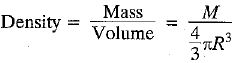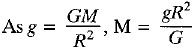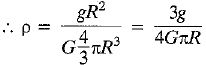Q.7. The earth is acted upon by gravitation of the Sun, even though it does not fail into the Sun. Why?
Ans: The earth remains in its circular orbit due to the gravitational force acting on it. Which provides centripetal force to the earth. Thus earth keeps rotating around Sun and does not fall into the Sun.

Q.8. How does the weight of an object vary with respect to mass and radius of the earth? In a hypothetical case, if the diameter of the earth becomes half of its present value and its mass becomes four times of its present value, then how would the weight of any object on the surface of the earth be affected?
Ans: W = mg and g = GM/R2
Hence W ∝ Mass of the earth (M)As M becomes 4 times and R is halved, W becomes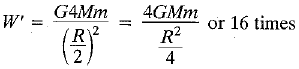Thus the weight becomes 16 times of earlier weight.

Q.9. How does the force of attraction between the two bodies depend upon their masses and distance between them? A student thought that two bricks tied together would fall faster than a single one under the action of gravity. Do you agree with this hypothesis or not? Comment.
Ans:  According to Newton’s law of gravitation,
F ∝ m1m2 (i.e. product of masses)
F ∝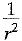(i.e. square of distance between them)
Time taken during free fall,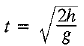Thus the hypothesis of student is incorrect as t is independent of mass of bricks.

Q.10. Two objects of masses m1 and m2 having the same size are dropped simultaneously from heights h1 and h2 respectively. Find out the ratio of time they would take in reaching the ground. Will this ratio remain the same if (i) one of the objects is hollow and the other one is solid and (ii) both of them are hollow, size remaining the same in each case. Give reason.
Ans: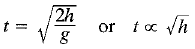Thus,
(i) If one object is hollow and the other is solid, the ratio of time to reach the ground remains the same, as.t is independent of masses.
(ii) The result is still unchanged as t depends only on height of fall.

Offer running on EduRev: Apply code STAYHOME200 to get INR 200 off on our premium plan EduRev Infinity!

## Science Class 9

143 videos|224 docs|135 tests

,

,

,

,

,

,

,

,

,

,

,

,

,

,

,

,

,

,

,

,

,

;